# Understanding Panoramic Resolution

This appendix discusses one of the most frequently misunderstood concepts in QuickTime VR––panoramic resolution––and the issues and questions that typically arise in any discussion of the topic.

## What Is Panoramic Resolution?

Panoramic resolution may be one of the least well-understood concepts in QuickTime VR. A number of questions arise in any discussion of the topic:

• If you want to measure the resolution of a panorama, how do you go about doing so?

• How do you compare the resolution of different kinds of panoramas?

• How does the resolution of a source image relate to that of a resultant panorama after stitching?

• How does the resolution of a fisheye image, for example, compare with that of a rectilinear image?

These questions can be answered by comparing the focal length or, equivalently, the angular pixel density of a QuickTime VR panorama.

### Defining Angular Pixel Density

Before going any further, it is important to define what is meant by angular pixel density. Typically, no image format is uniform in angular pixel density across the whole image.

Focal length is used as the basis of the definition. The focal length is defined as the distance to the imaging surface at the center of projection. This definition is consistent with that for perspective and fisheye lenses.

Focal length can be interpreted for various image formats in the following ways:

• For a perspective image, the distance to the imaging plane at the center.

• For a cubic panorama, the distance to the center of one of the faces.

• For a cylindircal panorama, the cylindrical radius (at the equator).

• For an equirectangular spherical panorama, the radius of the imaging sphere.

• For a fisheye image, the radius of the imaging sphere.

The pixel is used as the unit of measurement for image resolution and focal length. In these units, the focal length is identical to the angular pixel density at the center of projection. It is preferable to use the focal length to define panorama resolution because it is a parameter that is central to the mathematics of projection, and is well-defined for any panoramic image format.

Panorama resolution defined in terms of the focal length happens to be the minimum angular pixel density for the panorama formats considered here, when “square” pixels are used. This is not necessarily true for other panorama formats, though, such as annular formats.

### Panoramic Resolution in Pixels Per Degree

The units for focal length, when interpreted as angular pixel density, are pixels per radian.

It is more convenient to think of angular pixel density in terms of pixels per degree instead of pixels per radian, for several reasons:

• Degrees are used almost exclusively to quantify the measurement of angle, outside of mathematics; and

• The magnitude of currently published panoramas is of the order of a single decimal digit (1-7) when expressed in pixels per degree.

Thus, the following definition:

Panoramic resolution is the angular pixel density expressed in units of pixels per degree as determined by the focal length.

### Issues Involving Pixels and Focal Length

Still other issues arise: What do pixels, for example, have to do with focal length anyway? Focal length is traditionally measured in millimeters; however, on digital cameras, the focal length does not tell the whole story, because the digital camera specifications will say something like “it has a minimum 7 mm focal length, which is equivalent to a 35 mm focal length lens on a 35 mm camera”. The missing information is the target frame size, and using this information you can determine the field of view.

If you’re working typically with a camera that uses 35 mm film, you know that the frame size is 36 mm x 24 mm, as shown in the figure below.

Now if a 7 mm focal length on a digital camera is equivalent to a 35 mm focal length lens on a 35 mm camera, the CCD array in the camera must be 7.2 mm x 4.8 mm. The reason is that everything is in proportion.

### Computing Focal Length in Pixels

In a digital image, there are a given number of pixels. If you know the target frame size in millimeters, you can easily determine the number of pixels in a millimeter. If you also know the focal length of the lens used to make the picture, you can compute the focal length in pixels.

Now if you can figure out the focal length in pixels — what advantage does that offer you? As it happens, the focal length in pixels is the equivalent of the angular pixel density as expressed in pixels per radian. In this case, it is about 57 degrees (180/, to be exact). So if you convert from radians to degrees, you end up with an angular pixel density expressed in pixels per degree, and that unit of measurement means something. For example, a larger focal length means more pixels per panorama. And if you double the focal length, you double the number of pixels around the circumference.

In Table B-1, you can use the formulas in the last column of the table to compute the resolution in pixels per degree for some common panorama formats.

Table B-1 shows the formulas that you can use in order to compute the resolution in pixels per degree for some common panorama formats.

Table B-1  Resolution in Pixels per Degree for Common Panorama Formats

Dimension

Resolution (pixels/degree)

Perspective

small dimension in pixels (s)focal length in mm (f)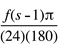Cubic

face dimension in pixels (w)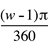Cylindrical

circumference in pixels (c)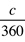Equirectangular Spherical

circumference in pixels (c)Round 180 degrees Fisheye

diameter in pixels (d)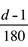Full Frame 180 degrees Fisheye

small dimension in pixels (s)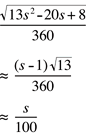Because it may be difficult to get an exact feel for the pixel economies with these formulas, Table B-2 is populated with the dimensions of various panorama formats at a resolution of 5.57 pixels per degree. This odd value of resolution was chosen to be that delivered by a 15 mm lens on a 35 mm camera, when the resulting image is 768 x 512 pixels.

Table B-2  Dimensions of various panorama formats in pixels

Panorama Image Format

Linear Pixels

Measure

Total Area in Pixels

Perspective (12 mm)

639

small dimension

Perspective (15 mm)

512

small dimension

Perspective (18 mm)

427

small dimension

Perspective (20 mm)

384

small dimension

Perspective (24 mm)

320

small dimension

Perspective (28 mm)

275

small dimension

Perspective (35 mm)

220

small dimension

Perspective (40 mm)

192

small dimension

Cubic

639

face dimension

2,449,926

Cylindrical

2005

circumference

2,447,675 @124.8FOV1,279,614 @90FOV

Equirectangular Spherical

2005

circumference

2,012,018

Single Round 180 Fisheye

1004

diameter

Double Round 180 Fisheye

1004

diameter

2,016,032 (1,583,388 without border)

Round 360 Fisheye

2005

diameter

4,020,025(3,157,320 without border)

Full Frame 180 Diagonal Fisheye

557

small dimension

The total number of pixels in the last column of the table includes border pixels needed to make each image rectangular. Since these can be chosen to compress well––for example, a solid color––these should add little to the size of the image. In the case of round fisheye images, a factor of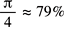can account for these. When determining pixel area, 180 vertical FOV is assumed for all except the cylindrical panoramas. For the perspective images, a 3:2 aspect ratio is assumed.

This allows you to compare efficiency of representation. Looking at memory usage, the equirectangular spherical format is the most efficient. Looking at compressed size, the double round 180 fisheye format is the most efficient, assuming that the black border compresses to nothing. The round 360 fisheye is the least efficient in either case, even when the border is removed.

It is common knowledge that the focal length in a perspective camera is the distance from the nodal point to the film or the CCD plane. Are there equivalent physical interpretations for the panorama formats? The answer is yes. For this, you need to use pixels per radian as the unit of measurement for the focal length. The physical interpretation of focal length is summarized in the Table B-3.

Table B-3  Physical interpretation of focal length

Type of Panorama

Focal Length defined as

Perspective image

Distance to imaging plane from nodal point

Cubic

Distance to face from center

Cylindrical